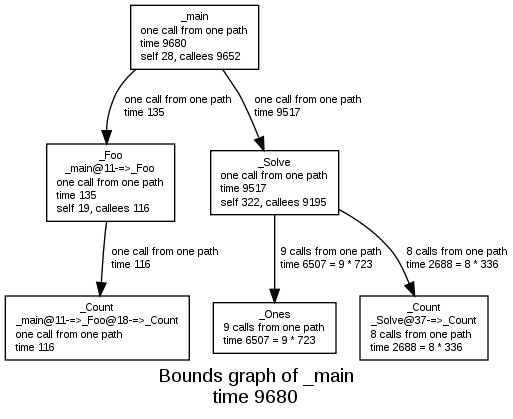### Call graph (bounds-graph form) from Bound-T analysis

The bounds-graph is a variant of the call graph that shows all the subprograms included in an analysis, all the call relationships between these subprograms, and all the WCET bounds computed for each subprogram in the worst-case execution scenario found by the analysis. In the bounds-graph form, if a subprogram has context-dependent WCET bounds (that is, different WCET bounds for different call paths) each context is shown as its own box. The subprogram appears in the drawing in as many boxes as it has context-dependent bounds.Note that in the drawing above, the subprogram Count is represented in three boxes, with different WCET bounds. All other subprograms have context-independent bounds and so appear in one box each.

Look here for the pure call graph with one box per subprogram.